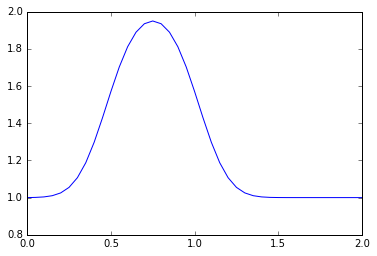Text provided under a Creative Commons Attribution license, CC-BY. All code is made available under the FSF-approved BSD-3 license. (c) Lorena A. Barba, Gilbert F. Forsyth 2017. Thanks to NSF for support via CAREER award #1149784.

# 12 steps to Navier–Stokes¶

You should have completed Steps 1 and 2 before continuing. This Jupyter notebook continues the presentation of the 12 steps to Navier–Stokes, the practical module taught in the interactive CFD class of Prof. Lorena Barba.

## Step 3: Diffusion Equation in 1-D¶

The one-dimensional diffusion equation is:

$$\frac{\partial u}{\partial t}= \nu \frac{\partial^2 u}{\partial x^2}$$

The first thing you should notice is that —unlike the previous two simple equations we have studied— this equation has a second-order derivative. We first need to learn what to do with it!

### Discretizing $\frac{\partial ^2 u}{\partial x^2}$¶

The second-order derivative can be represented geometrically as the line tangent to the curve given by the first derivative. We will discretize the second-order derivative with a Central Difference scheme: a combination of Forward Difference and Backward Difference of the first derivative. Consider the Taylor expansion of $u_{i+1}$ and $u_{i-1}$ around $u_i$:

$u_{i+1} = u_i + \Delta x \frac{\partial u}{\partial x}\bigg|_i + \frac{\Delta x^2}{2} \frac{\partial ^2 u}{\partial x^2}\bigg|_i + \frac{\Delta x^3}{3!} \frac{\partial ^3 u}{\partial x^3}\bigg|_i + O(\Delta x^4)$

$u_{i-1} = u_i - \Delta x \frac{\partial u}{\partial x}\bigg|_i + \frac{\Delta x^2}{2} \frac{\partial ^2 u}{\partial x^2}\bigg|_i - \frac{\Delta x^3}{3!} \frac{\partial ^3 u}{\partial x^3}\bigg|_i + O(\Delta x^4)$

If we add these two expansions, you can see that the odd-numbered derivative terms will cancel each other out. If we neglect any terms of $O(\Delta x^4)$ or higher (and really, those are very small), then we can rearrange the sum of these two expansions to solve for our second-derivative.

$u_{i+1} + u_{i-1} = 2u_i+\Delta x^2 \frac{\partial ^2 u}{\partial x^2}\bigg|_i + O(\Delta x^4)$

Then rearrange to solve for $\frac{\partial ^2 u}{\partial x^2}\bigg|_i$ and the result is:

$$\frac{\partial ^2 u}{\partial x^2}=\frac{u_{i+1}-2u_{i}+u_{i-1}}{\Delta x^2} + O(\Delta x^2)$$

### Back to Step 3¶

We can now write the discretized version of the diffusion equation in 1D:

$$\frac{u_{i}^{n+1}-u_{i}^{n}}{\Delta t}=\nu\frac{u_{i+1}^{n}-2u_{i}^{n}+u_{i-1}^{n}}{\Delta x^2}$$

As before, we notice that once we have an initial condition, the only unknown is $u_{i}^{n+1}$, so we re-arrange the equation solving for our unknown:

$$u_{i}^{n+1}=u_{i}^{n}+\frac{\nu\Delta t}{\Delta x^2}(u_{i+1}^{n}-2u_{i}^{n}+u_{i-1}^{n})$$

The above discrete equation allows us to write a program to advance a solution in time. But we need an initial condition. Let's continue using our favorite: the hat function. So, at $t=0$, $u=2$ in the interval $0.5\le x\le 1$ and $u=1$ everywhere else. We are ready to number-crunch!

In :
import numpy                 #loading our favorite library
from matplotlib import pyplot    #and the useful plotting library
%matplotlib inline

nx = 41
dx = 2 / (nx - 1)
nt = 20    #the number of timesteps we want to calculate
nu = 0.3   #the value of viscosity
dt = sigma * dx**2 / nu #dt is defined using sigma ... more later!

u = numpy.ones(nx)      #a numpy array with nx elements all equal to 1.
u[int(.5 / dx):int(1 / dx + 1)] = 2  #setting u = 2 between 0.5 and 1 as per our I.C.s

un = numpy.ones(nx) #our placeholder array, un, to advance the solution in time

for n in range(nt):  #iterate through time
un = u.copy() ##copy the existing values of u into un
for i in range(1, nx - 1):
u[i] = un[i] + nu * dt / dx**2 * (un[i+1] - 2 * un[i] + un[i-1])

pyplot.plot(numpy.linspace(0, 2, nx), u);For a careful walk-through of the discretization of the diffusion equation with finite differences (and all steps from 1 to 4), watch Video Lesson 4 by Prof. Barba on YouTube.

In :
from IPython.display import YouTubeVideo

from IPython.core.display import HTML